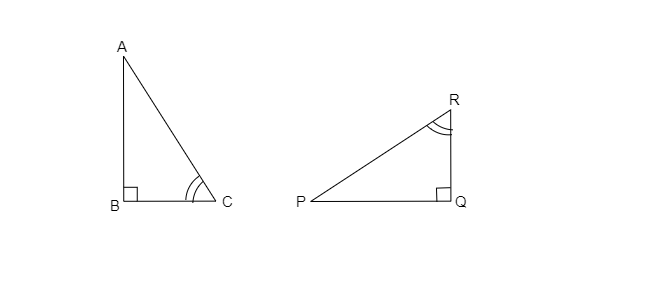# If ‚ąÜABC and ‚ąÜPQR are to be congruent, name one additional pair of corresponding parts. What criterion did you use?"

Given: ‚ąÜABC and ‚ąÜPQR are congruent in the given figure.

To do: To name one additional pair of corresponding parts.

Solution:

In $\triangle ABC$ and $\triangle PQR$

$\angle B=\angle Q=90^{\circ}$

$\angle C=\angle R$      [Given]

$\overline{BC}=\overline{QR}$     [Given]

Therefore, $\triangle ABC\cong\triangle PQR$    [ASA congruence]